### Example 84.8 Testing an Effect in a Logistic Regression Model

This example requests a two-sided test for the dose effect in a dose-response model (Whitehead, 1997, pp. 262–263). Consider the logistic regression model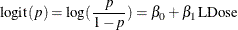where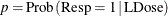is the response probability to be modeled for the binary response Resp and LDose = log( Dose +1) is the covariate. The dose levels are 0 for the control group, and they are 1, 3, and 6 for the three treatment groups.

Following the derivations in the section Test for a Parameter in the Logistic Regression Model in the chapter The SEQDESIGN Procedure, the required sample size can be derived from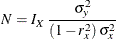where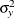is the variance of the response variable in the logistic regression model,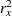is the proportion of variance of LDose explained by other covariates, and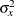is the variance of LDose.

Since LDose is the only covariate in the model,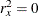. For a logistic model, the variancecan be estimated by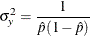where
is the estimated probability of the response variable Resp. Thus, the sample size can be computed as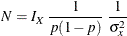The null hypothesis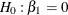corresponds to no treatment effect. Suppose that the alternative hypothesis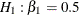is the reference improvement that should be detected at a 0.90 level.

Note that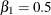corresponds to an odds ratio of 2 between the treatment group with dose level 3 and the control group. The log odds ratio between the two groups is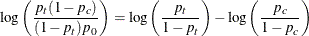which corresponds to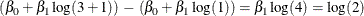If the same number of patients are assigned in each of the four groups, then the MLE of the variance of LDose is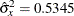. Further, if the response rate is 0.40, then the required sample size can be derived using the SAMPLESIZE statement in the SEQDESIGN procedure.

The following statements invoke the SEQDESIGN procedure and request a three-stage group sequential design for normally distributed data. The design has a null hypothesis of no treatment effectwith early stopping to reject the null hypothesis with a two-sided alternative hypothesis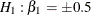.

ods graphics on;
proc seqdesign altref=0.5;
TwoSidedErrorSpending: design method=errfuncpow
method(loweralpha)=errfuncpow(rho=1)
method(upperalpha)=errfuncpow(rho=3)
nstages=3
stop=both;
samplesize model=logistic( prop=0.4 xvariance=0.5345);
ods output Boundary=Bnd_Dose;
run;
ods graphics off;


The ODS OUTPUT statement with the BOUNDARY=BND_DOSE option creates an output data set named BND_DOSE which contains the resulting boundary information for the subsequent sequential tests.

The Design Information table in Output 84.8.1 displays design specifications and derived statistics. Since the alternative reference is specified, the maximum information 47.22445 is derived.

The SEQDESIGN Procedure
Design: TwoSidedErrorSpending

Statistic Distribution Normal
Boundary Scale Standardized Z
Alternative Hypothesis Two-Sided
Early Stop Accept/Reject Null
Method Error Spending
Boundary Key Both
Alternative Reference 0.5
Number of Stages 3
Alpha 0.05
Beta (Lower) 0.1
Beta (Upper) 0.07871
Power (Lower) 0.9
Power (Upper) 0.92129
Max Information (Percent of Fixed Sample) 103.7223
Max Information 47.22445
Null Ref ASN (Percent of Fixed Sample) 79.47628
Lower Alt Ref ASN (Sample Size) 234.1646
Upper Alt Ref ASN (Sample Size) 270.4058

The Boundary Information table in Output 84.8.2 displays the information level, alternative reference, and boundary values at each stage. By default (or equivalently if you specify BOUNDARYSCALE=STDZ), the boundary values are displayed with the standardized Z statistic scale.

Output 84.8.2: Boundary Information

Boundary Information (Standardized Z Scale)
Null Reference = 0
_Stage_   Alternative Boundary Values
Information Level Reference Lower Upper
Proportion Actual N Lower Upper Alpha Beta Beta Alpha
1 0.3333 15.74148 122.7119 -1.98378 1.98378 -2.39398 . . 3.11302
2 0.6667 31.48297 245.4238 -2.80548 2.80548 -2.29380 -1.02812 0.91855 2.46193
3 1.0000 47.22445 368.1357 -3.43600 3.43600 -2.17479 -2.17479 1.98311 1.98311

With ODS Graphics enabled, a detailed boundary plot with the rejection and acceptance regions is displayed, as shown in Output 84.8.3.

Output 84.8.3: Boundary Plot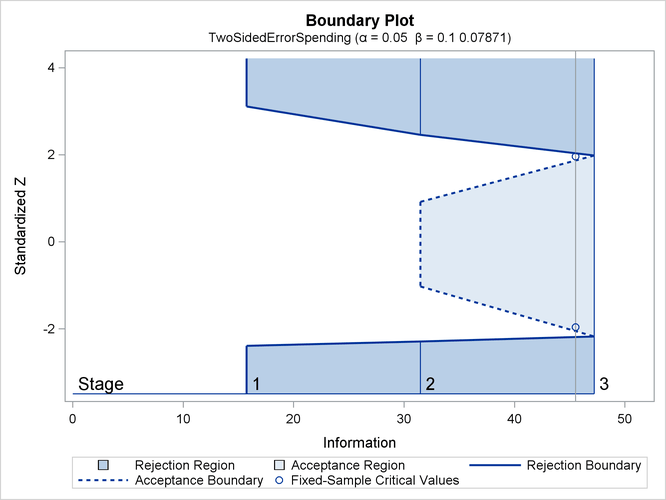With the SAMPLESIZE statement, the Sample Size Summary table in Output 84.8.4 displays the parameters for the sample size computation.

Output 84.8.4: Required Sample Size Summary

Sample Size Summary
Test Logistic Reg Parameter
Parameter 0.5
Proportion 0.4
X Variance 0.5345
R Square (X) 0
Max Sample Size 368.1357
Expected Sample Size (Null Ref) 282.0807
Expected Sample Size (Alt Ref) 270.4058

The Sample Sizes table in Output 84.8.5 displays the required sample sizes for the group sequential clinical trial.

Output 84.8.5: Required Sample Sizes

Sample Sizes (N)
Z Test for Logistic Regression Parameter
_Stage_ Fractional N Ceiling N
N Information N Information
1 122.71 15.7415 123 15.7784
2 245.42 31.4830 246 31.5569
3 368.14 47.2245 369 47.3353

That is, 123 new patients are needed in each stage and the number is rounded up to 124 for each stage to have a multiple of four for the four dose levels in the trial. Note that since the sample sizes are derived from an estimated response probability and are rounded up, the actual information levels might not match the corresponding target information levels.

Output 84.8.6 lists the first 10 observations of the trial data.

Output 84.8.6: Clinical Trial Data

 First 10 Obs in the Trial Data

Obs Resp Dose LDose
1 0 0 0.00000
2 0 1 0.69315
3 1 3 1.38629
4 1 6 1.94591
5 1 0 0.00000
6 1 1 0.69315
7 1 3 1.38629
8 1 6 1.94591
9 0 0 0.00000
10 0 1 0.69315

The following statements use the LOGISTIC procedure to estimate the slope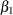and its associated standard error at stage 1:

proc logistic data=Dose_1;
model Resp(event='1')= LDose;
ods output ParameterEstimates=Parms_Dose1;
run;


The following statements create and display (in Output 84.8.7) the input data set that contains slopeand its associated standard error for the SEQTEST procedure:

data Parms_Dose1;
set Parms_Dose1;
if Variable='LDose';
_Scale_='MLE';
_Stage_= 1;
keep _Scale_ _Stage_ Variable Estimate StdErr;
run;

proc print data=Parms_Dose1;
title 'Statistics Computed at Stage 1';
run;


Output 84.8.7: Statistics Computed at Stage 1

 Statistics Computed at Stage 1

Obs Variable Estimate StdErr _Scale_ _Stage_
1 LDose 0.5741 0.2544 MLE 1

The following statements invoke the SEQTEST procedure to test for early stopping at stage 1:

ods graphics on;
proc seqtest Boundary=Bnd_Dose
Parms(testvar=LDose)=Parms_Dose1
order=mle
boundaryscale=mle
;
ods output Test=Test_Dose1;
run;
ods graphics off;


The BOUNDARY= option specifies the input data set that provides the boundary information for the trial at stage 1, which was generated in the SEQDESIGN procedure. The PARMS=PARMS_DOSE1 option specifies the input data set PARMS_DOSE1 that contains the test statistic and its associated standard error at stage 1, and the TESTVAR=LDOSE option identifies the test variable LDOSE in the data set.

If the computed information level for stage 1 is not the same as the value provided in the BOUNDARY= data set, the INFOADJ=PROP option (which is the default) proportionally adjusts the information levels at future interim stages from the levels provided in the BOUNDARY= data set. The ORDER=MLE option uses the MLE ordering to derive the p-value, the unbiased median estimate, and the confidence limits for the regression slope estimate.

The ODS OUTPUT statement with the TEST=TEST_DOSE1 option creates an output data set named TEST_DOSE1 which contains the updated boundary information for the test at stage 1. The data set also provides the boundary information that is needed for the group sequential test at the next stage.

The Design Information table in Output 84.8.8 displays design specifications. By default (or equivalently if you specify BOUNDARYKEY=ALPHA), the boundary values are modified for the new information levels to maintain the Type Ilevel. The maximum information remains the same as the design stored in the BOUNDARY= data set, but the derived Type II error probabilityand power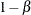are different because of the new information levels.

The SEQTEST Procedure

BOUNDARY Data Set WORK.BND_DOSE
Data Set WORK.PARMS_DOSE1
Statistic Distribution Normal
Boundary Scale MLE
Alternative Hypothesis Two-Sided
Early Stop Accept/Reject Null
Number of Stages 3
Alpha 0.05
Beta (Lower) 0.09992
Beta (Upper) 0.07871
Power (Lower) 0.90008
Power (Upper) 0.92129
Max Information (Percent of Fixed Sample) 103.7231
Max Information 47.2244524
Null Ref ASN (Percent of Fixed Sample) 79.45049
Lower Alt Ref ASN (Percent of Fixed Sample) 66.05269
Upper Alt Ref ASN (Percent of Fixed Sample) 76.24189

The Test Information table in Output 84.8.9 displays the boundary values for the test statistic with the specified MLE scale.

Output 84.8.9: Sequential Tests

Test Information (MLE Scale)
Null Reference = 0
_Stage_   Alternative Boundary Values Test
Information Level Reference Lower Upper LDose
Proportion Actual Lower Upper Alpha Beta Beta Alpha Estimate Action
1 0.3272 15.45062 -0.50000 0.50000 -0.61078 . . 0.79337 0.57409 Continue
2 0.6636 31.33753 -0.50000 0.50000 -0.40974 -0.18169 0.16222 0.44033 .
3 1.0000 47.22445 -0.50000 0.50000 -0.31633 -0.31633 0.28860 0.28860 .

The information level at stage 1 is derived from the standard error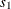in the PARMS= data set,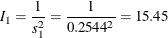With the INFOADJ=PROP option (which is the default), the information level at stage 2 is derived proportionally from the observed information at stage 1 and the information levels in the BOUNDARY= data set. At stage 1, theboundary values are missing and there is no early stopping to accept. The MLE statistic 0.57409 is between the lower and upperboundaries, so the trial continues to the next stage.

With ODS Graphics enabled, a boundary plot with the boundary values and test statistics is displayed, as shown in Output 84.8.10. As expected, the test statistic is in the continuation region below the upper alpha boundary.

Output 84.8.10: Sequential Test Plot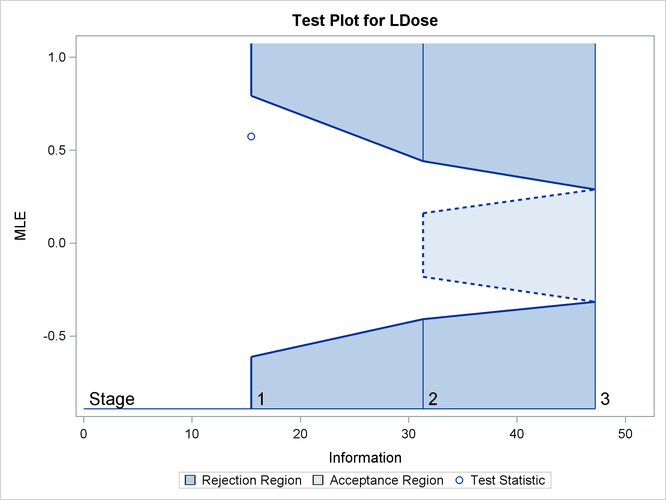The following statements use the LOGISTIC procedure to estimate the slopeand its associated standard error at stage 2:

proc logistic data=dose_2;
model Resp(event='1')=LDose;
ods output ParameterEstimates=Parms_Dose2;
run;


The following statements create and display (in Output 84.8.11) the input data set that contains slopeand its associated standard error at stage 2 for the SEQTEST procedure:

data Parms_Dose2;
set Parms_Dose2;
if Variable='LDose';
_Scale_='MLE';
_Stage_= 2;
keep _Scale_ _Stage_ Variable Estimate StdErr;
run;

proc print data=Parms_Dose2;
title 'Statistics Computed at Stage 2';
run;


Output 84.8.11: Statistics Computed at Stage 2

 Statistics Computed at Stage 2

Obs Variable Estimate StdErr _Scale_ _Stage_
1 LDose 0.5213 0.1788 MLE 2

The following statements invoke the SEQTEST procedure to test for early stopping at stage 2:

ods graphics on;
proc seqtest Boundary=Test_Dose1
Parms(Testvar=LDose)=Parms_Dose2
order=mle
boundaryscale=mle
rci
plots=rci
;
ods output Test=Test_Dose2;
run;
ods graphics off;


The BOUNDARY= option specifies the input data set that provides the boundary information for the trial at stage 2, which was generated by the SEQTEST procedure at the previous stage. The PARMS= option specifies the input data set that contains the test statistic and its associated standard error at stage 2, and the TESTVAR= option identifies the test variable in the data set.

The ORDER=MLE option uses the MLE ordering to derive the p-value, unbiased median estimate, and confidence limits for the regression slope estimate.

The ODS OUTPUT statement with the TEST=TEST_DOSE2 option creates an output data set named TEST_DOSE2 which contains the updated boundary information for the test at stage 2. The data set also provides the boundary information that is needed for the group sequential test at the next stage.

The Design Information table in Output 84.8.12 displays design specifications. By default (or equivalently if you specify BOUNDARYKEY=ALPHA), the boundary values are modified for the new information levels to maintain the Type Ilevel.

The SEQTEST Procedure

BOUNDARY Data Set WORK.TEST_DOSE1
Data Set WORK.PARMS_DOSE2
Statistic Distribution Normal
Boundary Scale MLE
Alternative Hypothesis Two-Sided
Early Stop Accept/Reject Null
Number of Stages 3
Alpha 0.05
Beta (Lower) 0.0999
Beta (Upper) 0.07871
Power (Lower) 0.9001
Power (Upper) 0.92129
Max Information (Percent of Fixed Sample) 103.7227
Max Information 47.2244524
Null Ref ASN (Percent of Fixed Sample) 79.44086
Lower Alt Ref ASN (Percent of Fixed Sample) 66.04641
Upper Alt Ref ASN (Percent of Fixed Sample) 76.22739

The information is derived from the standard error associated with the slope estimate at the final stage and is larger than the target level. The derived Type II error probabilityand powerare different because of the new information levels.

The Test Information table in Output 84.8.13 displays the boundary values for the test statistic with the specified MLE scale. The information levels are derived from the standard errors in the PARMS= data set. At stage 2, the slope estimate 0.52128 is larger than 0.44091, the upperboundary value, the trial stops to reject the null hypothesis of no treatment effect.

Output 84.8.13: Sequential Tests

Test Information (MLE Scale)
Null Reference = 0
_Stage_   Alternative Boundary Values Test
Information Level Reference Lower Upper LDose
Proportion Actual Lower Upper Alpha Beta Beta Alpha Estimate Action
1 0.3272 15.45062 -0.50000 0.50000 -0.61078 . . 0.79337 0.57409 Continue
2 0.6624 31.28346 -0.50000 0.50000 -0.41028 -0.18112 0.16166 0.44091 0.52128 Reject Null
3 1.0000 47.22445 -0.50000 0.50000 -0.31628 -0.31628 0.28861 0.28861 .

With ODS Graphics enabled, a boundary plot with the boundary values and test statistics is displayed, as shown in Output 84.8.14. As expected, the test statistic is above the upperboundary in the upper rejection region at stage 2.

Output 84.8.14: Sequential Test Plot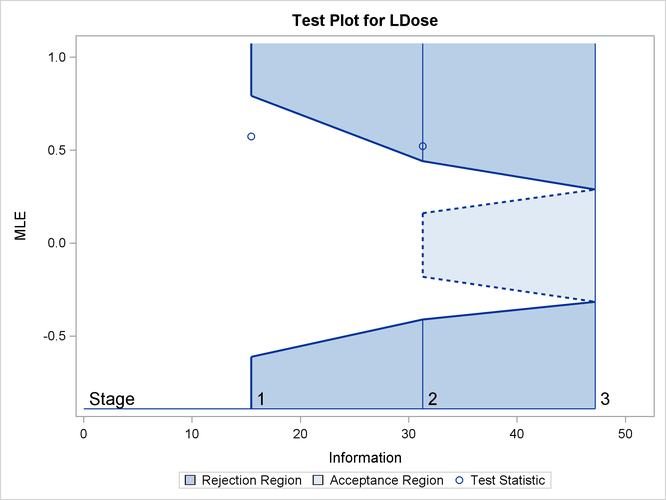After a trial is stopped, the Parameter Estimates table in Output 84.8.15 displays the stopping stage, parameter estimate, unbiased median estimate, confidence limits, and the p-value under the null hypothesis.

Output 84.8.15: Parameter Estimates

Parameter Estimates
MLE Ordering
Parameter Stopping
Stage
MLE p-Value for
H0:Parm=0
Median
Estimate
95% Confidence Limits
LDose 2 0.521275 0.0050 0.502647 0.15745 0.85154

With the ORDER=MLE option, the MLE ordering is used to compute the p-value, unbiased median estimate, and confidence limits. As expected, the p-value 0.005 is significant at the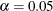level and the confidence interval does not contain the null reference zero.

With the RCI option, the Repeated Confidence Intervals table in Output 84.8.16 displays repeated confidence intervals for the parameter. For a two-sided test, since the rejection lower repeated confidence limit 0.0804 is greater than the null reference zero, the trial is stopped to reject the hypothesis.

Output 84.8.16: Repeated Confidence Intervals

Repeated Confidence Intervals
_Stage_ Information
Level
Parameter
Estimate
Rejection Boundary Acceptance Boundary
Lower 97.5% Repeated CL Upper 97.5% Repeated CL Lower 90.01% Repeated CL Upper 92.13% Repeated CL
1 15.4506 0.57409 -0.2193 1.1849 . .
2 31.2835 0.52128 0.0804 0.9316 0.2024 0.8596

With the PLOTS=RCI option, the Repeated Confidence Intervals Plot displays repeated confidence intervals for the parameter, as shown in Output 84.8.17. It shows that the null reference zero is inside the rejection repeated confidence interval at stage 1 but outside the rejection repeated confidence interval at stage 2. This implies that the study stops at stage 2 to reject the hypothesis.

Output 84.8.17: Repeated Confidence Intervals Plot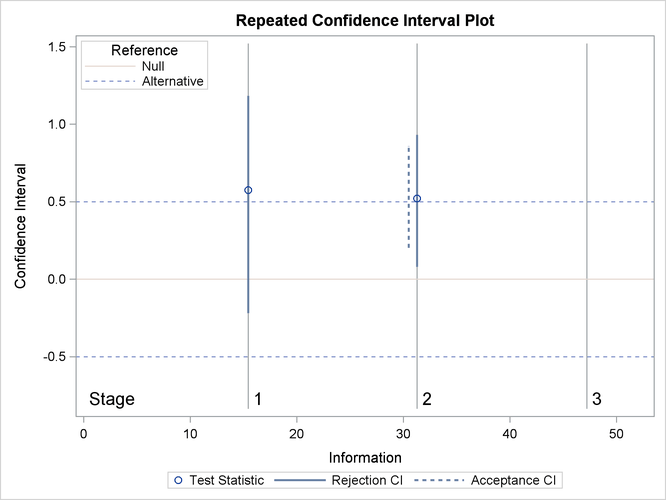Note that the hypothesis is accepted if at any stage, the acceptance repeated confidence interval falls within the interval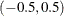of the alternative references.